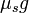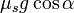# Traction

## Definition

Traction refers to the maximum possible value of friction force between a car and the road/ground on which it is running, for which the no slip condition is maintained, i.e., the wheels (of the tires) keep rolling.

It thus represents the maximum value of force that can be applied to accelerate or decelerate the car while maintaining the no slip condition.

## Value of traction

### On a horizontal road ignoring downforce

If we assume the Coulomb model of friction, then, on a horizontal road, we have:

Traction = Limiting coefficient of static friction (between the tires and the road)$\times$ Weight of the car.

If the limiting coefficient of static friction is$\mu_s$, the maximum achievable magnitude of acceleration while preserving the no slip condition is (independent of the mass of the car):$\mu_sg$

Here$g$ denotes the value of acceleration due to gravity.

In particular, the more slippery a surface, the lower the value of$\mu_s$, and the lower the maximum value of traction that can be achieved on the surface.

### On an incline

If$\alpha$ is the angle of the incline, the maximum possible traction along the incline (whether going up or down the incline) is$\mu_smg\cos \alpha$ where$m$ is the mass of the vehicle. The maximum possible magnitude of acceleration is thus$\mu_sg\cos \alpha$.

What this means is that along an incline, for the same pair of surfaces, the traction that can be achieved is less. In other words, it is harder to accelerate or decelerate a vehicle up or down an incline while making sure that the no slip condition is preserved.